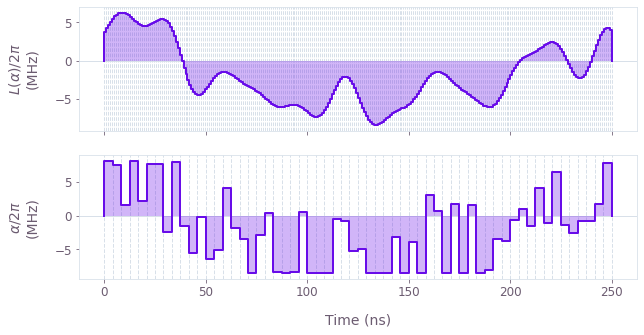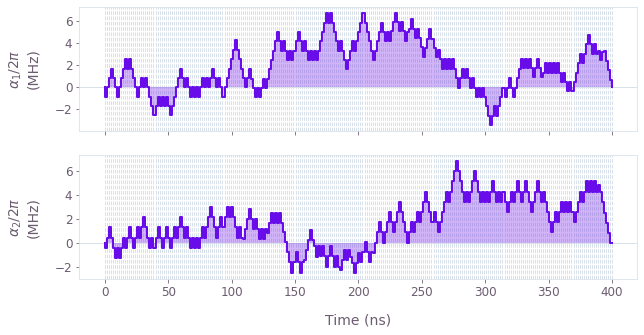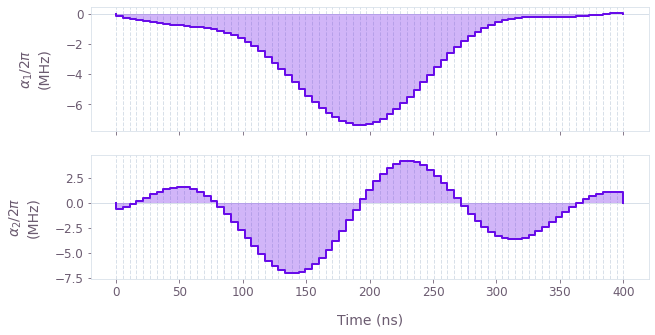Get the notebook

# How to add smoothing and band-limits to optimized controls

Incorporate smoothing of optimized waveforms

Boulder Opal exposes a highly-flexible optimization engine for general-purpose gradient-based optimization. It can be directly applied to model-based control optimization for arbitrary-dimensional quantum systems. In general the optimizer will employ whatever freedom it is given, including infinite-bandwidth transitions between time-slices of a piecewise-constant signal. However, such controls will not generally be faithfully reproduced on hardware, causing a challenge in realizing high-fidelity controls. However, by incorporating smoothing into the optimization routine we can ensure that the outputs of an optimization are well reproduced in hardware.

In this user guide we introduce three different techniques for creating smooth or band-limited optimized controls: incorporating linear time-invariant filters into an optimization; bounding slew-rates in controls; smoothing and discretizing controls. To learn the basics about control optimization, you can follow our robust optimization tutorial.

## Summary workflow

### 1. Define smoothing constraint in computational graph

The flexible Boulder Opal optimization engine expresses all optimization problems as data flow graphs, which describe how optimization variables (variables that can be tuned by the optimizer) are transformed into the cost function (the objective that the optimizer attempts to minimize).

In order to add smoothing you can pursue several different methods:

#### Filtering control signals

One can achieve tight frequency-domain control over the spectral content of a waveform using filtering. The technique we use here is to pass the controls through a linear time-invariant filter in order to enforce explicit bandwidth limits. To implement this behavior, we can create optimizable signals as usual, and then use the graph.utils.filter_and_resample_pwc node from the utils toolkit in Boulder Opal to generate the filtered piecewise-constant (PWC) signal.

graph.utils is part of the Boulder Opal Toolkits which are currently in beta phase of development. Breaking changes may be introduced.

The effect of these filters is to transform the control signals before they reach the quantum system, via convolution with the filter impulse response. Failing to take the filters into account during the optimization can lead to poor results, since in that case the system used for the optimization does not accurately model reality.

#### Bounding slew rates

A simple alternative is to constrain the rate of change of a signal between timesteps. To implement a bounded slew rate, you create signal values using a Boulder Opal graph operation, graph.anchored_difference_bounded_variables. This function produces values that are constrained to satisfy the slew rate requirement, and in addition are anchored to zero at the start and end of the gate.

#### Discretizing smoothed waveforms

An alternative approach to band limitation is to apply a filter that removes the higher frequencies from the signal, and then convert the signal again into a piecewise-constant pulse. If we additionally multiply the values of the signal by an envelope function that goes to zero at its extremities, we effectively anchor the beginning and the end of the pulse at zero.

### 2. Execute graph-based optimization

With the graph object created, an optimization can be run using the qctrl.functions.calculate_optimization function. The cost, the outputs, and the graph must be provided. The function returns the results of the optimization. Note that this example code block uses naming that should be replaced with the naming used in your graph.

## Worked example: Smoothing control pulses using linear filters

In this section we present examples showing how linear time-invariant filters may be incorporated into an optimization. To exemplify the use of filters, we consider a basic single-qubit system described by the Hamiltonian:

\begin{align} H(t) &= \frac{1}{2} L(\alpha)(t)\sigma_{x} + \beta(t) \sigma_{z} , \end{align}

where $\alpha(t)$ is a real time-dependent pulse, $\beta(t)$ is a small, slowly-varying stochastic dephasing noise process, and $L$ is the filter applied to the $\alpha(t)$ pulse. The effect of the filter is to transform the control signal before it reaches the quantum system, via convolution with the filter impulse response.

In this example, we use an in-built Boulder Opal function to produce the sinc filter to smooth the control pulse. From the optimizations we output two sets of signals: the raw piecewise-constant signal and its smoothed version. The latter is the filtered signal that actually reaches the quantum system and performs the optimized gate.

import matplotlib.pyplot as plt
import numpy as np
from qctrl import Qctrl
from qctrlvisualizer import plot_controls

# Starting a session with the API
qctrl = Qctrl()

# Define physical constraints
alpha_max = 2 * np.pi * 8.5e6  # rad/s
sinc_cutoff_frequency = 2 * np.pi * 48e6  # rad/s
segment_count = 60
duration = 250e-9  # s

# Create graph object
graph = qctrl.create_graph()

# Create alpha(t) signal
alpha = graph.utils.real_optimizable_pwc_signal(
segment_count=segment_count,
duration=duration,
maximum=alpha_max,
minimum=-alpha_max,
name="$\\alpha$",
)

# Create filtered signal
rediscretized_alpha = graph.utils.filter_and_resample_pwc(
pwc=alpha,
cutoff_frequency=sinc_cutoff_frequency,
segment_count=256,
name="$L(\\alpha)$",
)

# Create control term
control_sigma_x = rediscretized_alpha * graph.pauli_matrix("X") / 2

# Create dephasing noise term
dephasing = graph.pauli_matrix("Z") / duration

# Create infidelity
infidelity = graph.infidelity_pwc(
hamiltonian=control_sigma_x,
target=graph.target(graph.pauli_matrix("X")),
noise_operators=[dephasing],
name="infidelity",
)

# Run the optimization
result = qctrl.functions.calculate_optimization(
graph=graph,
cost_node_name="infidelity",
output_node_names=["$\\alpha$", "$L(\\alpha)$"],
)

print(f"Optimized cost: {result.cost:.3e}")

# Visualize controls
plot_controls(plt.figure(), result.output)
plt.show()

Your task calculate_optimization (action_id="1164545") has started. You can use the qctrl.get_result method to retrieve previous results.
Optimized cost: 1.185e-12Unfiltered (top) and filtered (bottom) control amplitudes as a function of time.

In this example, we re-discretized the filtered pulse to plot it as a finely-grained piecewise-constant plot that can be compared to its unfiltered counterpart. The re-discretization can be skipped if plotting the filtered controls is not desired.

## Worked example: Band-limited pulses with bounded slew rates

Next we show how to optimize a system in which the rates of change of the controls are limited. Using this constraint can help to ensure that optimized controls can be reliably implemented on physical hardware (which may be subject to bandwidth limits). We consider a standard single-qubit system subject to dephasing noise:

\begin{align} H(t) &= \frac{1}{2} \alpha_1(t)\sigma_{x} + \frac{1}{2} \alpha_2(t) \sigma_{z} + \beta(t) \sigma_{z} , \end{align}

where $\alpha_1(t)$ and $\alpha_2(t)$ are real time-dependent pulse and $\beta(t)$ is a small, slowly-varying stochastic dephasing noise process. In this case, we enforce a maximum slew rate constraint on $\alpha_1(t)$ and $\alpha_2(t)$, to cap the variation between adjacent segment values.

# Define physical constraints
alpha_max = 2 * np.pi * 8.5e6  # rad/s
max_slew_rate = alpha_max / 10
segment_count = 250
duration = 400e-9  # s

# Create graph object
graph = qctrl.create_graph()

# Create alpha_1(t) signal
alpha_1_values = graph.anchored_difference_bounded_variables(
count=segment_count,
lower_bound=-alpha_max,
upper_bound=alpha_max,
difference_bound=max_slew_rate,
)
alpha_1 = graph.pwc_signal(values=alpha_1_values, duration=duration, name="$\\alpha_1$")

# Create alpha_2(t) signal
alpha_2_values = graph.anchored_difference_bounded_variables(
count=segment_count,
lower_bound=-alpha_max,
upper_bound=alpha_max,
difference_bound=max_slew_rate,
)
alpha_2 = graph.pwc_signal(values=alpha_2_values, duration=duration, name="$\\alpha_2$")

# Create dephasing noise term
dephasing = graph.pauli_matrix("Z") / duration

# Create target
target_operator = graph.target(operator=graph.pauli_matrix("Y"))

# Create infidelity
infidelity = graph.infidelity_pwc(
hamiltonian=(alpha_1 * graph.pauli_matrix("X") + alpha_2 * graph.pauli_matrix("Z"))
/ 2,
target=target_operator,
noise_operators=[dephasing],
name="infidelity",
)

# Run the optimization
result = qctrl.functions.calculate_optimization(
graph=graph,
cost_node_name="infidelity",
output_node_names=["$\\alpha_1$", "$\\alpha_2$"],
)

print(f"Optimized cost: {result.cost:.3e}")

# Plot the optimized controls
plot_controls(plt.figure(), result.output)
plt.show()

Your task calculate_optimization (action_id="1164557") has started. You can use the qctrl.get_result method to retrieve previous results.
Optimized cost: 1.190e-12Pulse amplitudes obtained from a band-limited optimization with bounded-slew rates for $\alpha_1(t)$ (top) and $\alpha_2(t)$ (bottom).

## Worked example: Band-limited pulses via discretized smooth functions

In the code cell below, we use this approach to optimize a pulse in the same physical setting as the previous subsection.

# Define physical constraints
alpha_max = 2 * np.pi * 8.5e6  # Hz
segment_count = 75
duration = 400e-9  # s
cutoff_frequency = segment_count / duration / 4

# Create graph object
graph = qctrl.create_graph()

envelope_signal = graph.pulses.cosine_pulse_pwc(
duration=duration, segment_count=segment_count, amplitude=1.0
)

# Create alpha_1(t) signal
alpha_1_values = graph.utils.real_optimizable_pwc_signal(
segment_count=segment_count,
minimum=-alpha_max,
maximum=alpha_max,
duration=duration,
)

alpha_1 = alpha_1_values * envelope_signal

# Create alpha_2(t) signal
alpha_2_values = graph.utils.real_optimizable_pwc_signal(
segment_count=segment_count,
minimum=-alpha_max,
maximum=alpha_max,
duration=duration,
)

alpha_2 = alpha_2_values * envelope_signal

# Remove higher frequencies
rediscretized_alpha_1 = graph.utils.filter_and_resample_pwc(
pwc=alpha_1,
cutoff_frequency=cutoff_frequency,
segment_count=segment_count,
name="$\\alpha_1$",
)
rediscretized_alpha_2 = graph.utils.filter_and_resample_pwc(
pwc=alpha_2,
cutoff_frequency=cutoff_frequency,
segment_count=segment_count,
name="$\\alpha_2$",
)

# Create control terms
control_x = rediscretized_alpha_1 * graph.pauli_matrix("X") / 2
control_z = rediscretized_alpha_2 * graph.pauli_matrix("Z") / 2

# Create dephasing noise term
dephasing = graph.pauli_matrix("Z") / duration

# Create infidelity
infidelity = graph.infidelity_pwc(
hamiltonian=(control_x + control_z),
target=graph.target(graph.pauli_matrix("Y")),
noise_operators=[dephasing],
name="infidelity",
)

# Run the optimization
result = qctrl.functions.calculate_optimization(
graph=graph,
cost_node_name="infidelity",
output_node_names=["$\\alpha_1$", "$\\alpha_2$"],
)

print(f"Optimized cost: {result.cost:.3e}")

# Visualize optimized pulses
plot_controls(plt.figure(), result.output)
plt.show()

Your task calculate_optimization (action_id="1164614") has started. You can use the qctrl.get_result method to retrieve previous results.
Optimized cost: 4.545e-12Pulse amplitudes obtained from a band-limited optimization with anchored filtered pulses for $\alpha_1(t)$ (top) and $\alpha_2(t)$ (bottom).

This notebook was run using the following package versions. It should also be compatible with newer versions of the Q-CTRL Python package.

Package version
Python 3.9.12
matplotlib 3.5.1
numpy 1.21.5
scipy 1.7.3
qctrl 19.1.0
qctrlcommons 17.1.1
qctrltoolkit 1.5.0
qctrlvisualizer 3.2.1

## Next up

Continue learning about Boulder Opal

### How to optimize controls with nonlinear dependences

Incorporate nonlinear Hamiltonian dependences on control signals